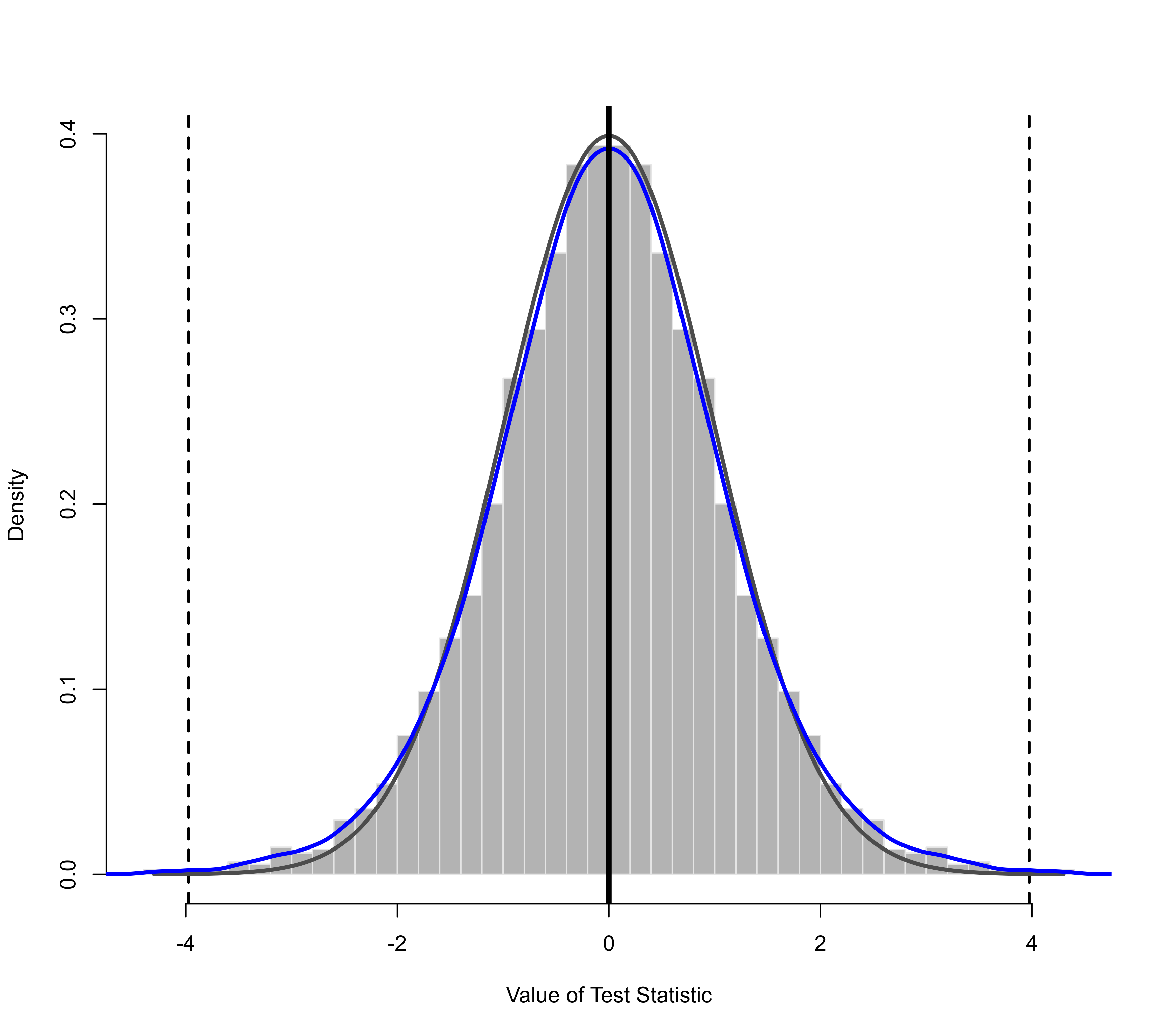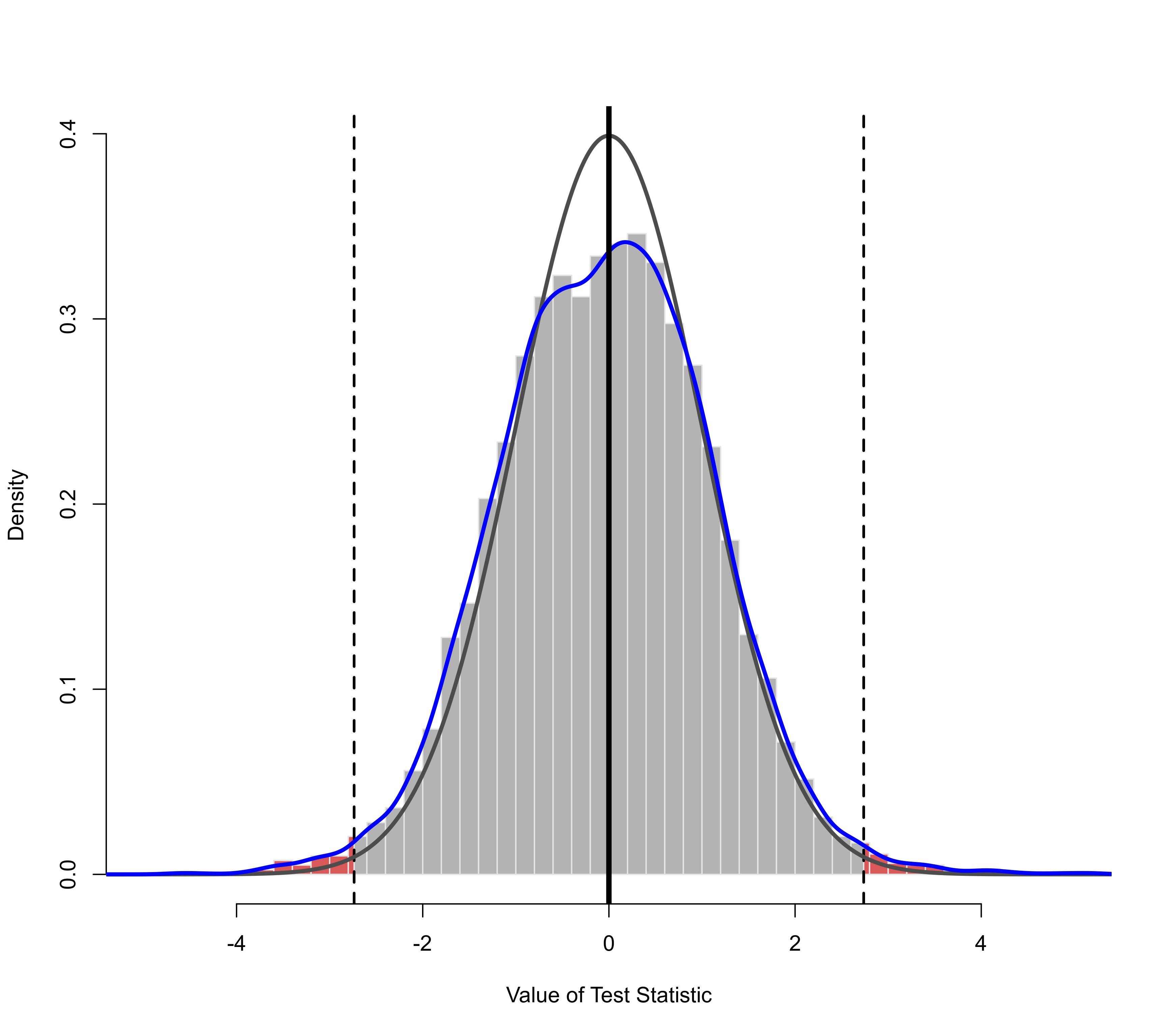Plot method for objects of class "permutest.rma.uni".

# S3 method for permutest.rma.uni
plot(x, beta, alpha, QM=FALSE, QS=FALSE,
breaks="Scott", freq=FALSE, col="lightgray", border=NULL,
col.out=rgb(1,0,0,0.5), col.ref="black", col.density="blue",
adjust=1, lwd=c(2,0,0,4), layout, ...)

## Arguments

x

an object of class "permutest.rma.uni" obtained with permutest.

beta

optional vector of indices to specify which (location) coefficients should be plotted.

alpha

optional vector of indices to specify which scale coefficients should be plotted. Only relevant for location-scale models (see rma.uni).

QM

logical to specify whether the permutation distribution of the omnibus test of the (location) coefficients should be plotted (the default is FALSE).

QS

logical to specify whether the permutation distribution of the omnibus test of the scale coefficients should be plotted (the default is FALSE). Only relevant for location-scale models (see rma.uni).

breaks

argument to be passed on to the corresponding argument of hist to set (the method for determining) the (number of) breakpoints.

freq

logical to indicate whether frequencies or probability densities should be plotted (the default is FALSE to plot densities).

col

character string to specify the color for the bars (the default is a light gray).

border

character string to specify the color for the border around the bars (the default is NULL to use the standard foreground color).

col.out

character string to specify the color for the bars that are more extreme than the observed test statistic (the default is a semi-transparent shade of red).

col.ref

character string to specify the color of the theoretical reference/null distribution that is superimposed on top of the histogram (the default is black).

col.density

character string to specify the color of the kernel density estimate of the permutation distribution that is superimposed on top of the histogram (the default is blue).

numeric value to be passed on to the corresponding argument of density (for adjusting the bandwidth of the kernel density estimate).

lwd

numeric vector to specify the width of the vertical lines corresponding to the value of the observed test statistic, of the reference/null distribution, of the density estimate, and of the vertical line at 0. Note: by default, the reference/null distribution and the density estimate have a line width of 0 and are therefore not plotted.

layout

optional vector of two numbers to specify the number of rows and columns for the layout of the figure.

...

other arguments.

## Details

The function plots the permutation distribution(s) of the model coefficient(s) as histograms.

For models with moderators, one can choose via argument beta which coefficients to plot (by default, all permutation distributions except that of the intercept are plotted). One can also choose to plot the permutation distribution of the omnibus test of the model coefficients (by setting QM=TRUE).

Arguments breaks, freq, col, and border are passed on to the hist function for the plotting.

Argument col.out is used to specify the color for the bars that are more extreme than the observed test statistic. The p-value of a permutation test corresponds to the area of these bars.

One can superimpose the theoretical reference/null distribution on top of the histogram (i.e., the distribution as assumed by the model). The p-value for the standard (i.e., non-permutation) test is the area that is more extreme than the observed test statistic under this reference/null distribution.

A kernel density estimate of the permutation distribution can also be superimposed on top of the histogram (as a smoothed representation of the permutation distribution).

Note that the theoretical reference/null distribution and the kernel density estimate of the permutation distribution are only shown when setting the line width for these elements greater than 0 via the lwd argument.

For location-scale models (see rma.uni for details), one can also use arguments alpha and QS to specify which scale coefficients to plot and whether to also plot the permutation distribution of the omnibus test of the scale coefficients (by setting QS=TRUE).

## Author

Wolfgang Viechtbauer wvb@metafor-project.org https://www.metafor-project.org

permutest.rma.uni for the function to create permutest.rma.uni objects.

## Examples

### calculate log risk ratios and corresponding sampling variances
dat <- escalc(measure="RR", ai=tpos, bi=tneg, ci=cpos, di=cneg, data=dat.bcg)

### random-effects model
res <- rma(yi, vi, data=dat)
res
#>
#> Random-Effects Model (k = 13; tau^2 estimator: REML)
#>
#> tau^2 (estimated amount of total heterogeneity): 0.3132 (SE = 0.1664)
#> tau (square root of estimated tau^2 value):      0.5597
#> I^2 (total heterogeneity / total variability):   92.22%
#> H^2 (total variability / sampling variability):  12.86
#>
#> Test for Heterogeneity:
#> Q(df = 12) = 152.2330, p-val < .0001
#>
#> Model Results:
#>
#> estimate      se     zval    pval    ci.lb    ci.ub
#>  -0.7145  0.1798  -3.9744  <.0001  -1.0669  -0.3622  ***
#>
#> ---
#> Signif. codes:  0 ‘***’ 0.001 ‘**’ 0.01 ‘*’ 0.05 ‘.’ 0.1 ‘ ’ 1
#>

# \dontrun{
### permutation test (exact)
set.seed(1234) # for reproducibility
permres <- permutest(res, exact=TRUE)
#> Running 8192 iterations for an exact permutation test.
permres
#>
#> Model Results:
#>
#> estimate      se     zval    pval¹    ci.lb    ci.ub
#>  -0.7145  0.1798  -3.9744  0.0015   -1.0669  -0.3622  **
#>
#> ---
#> Signif. codes:  0 ‘***’ 0.001 ‘**’ 0.01 ‘*’ 0.05 ‘.’ 0.1 ‘ ’ 1
#>
#> 1) p-value based on permutation testing
#>

### plot of the permutation distribution
### dashed horizontal line: the observed value of the test statistic (in both tails)
### black curve: standard normal density (theoretical reference/null distribution)
### blue curve: kernel density estimate of the permutation distribution
plot(permres, lwd=c(2,3,3,4))### mixed-effects model with two moderators (absolute latitude and publication year)
res <- rma(yi, vi, mods = ~ ablat + year, data=dat)
res
#>
#> Mixed-Effects Model (k = 13; tau^2 estimator: REML)
#>
#> tau^2 (estimated amount of residual heterogeneity):     0.1108 (SE = 0.0845)
#> tau (square root of estimated tau^2 value):             0.3328
#> I^2 (residual heterogeneity / unaccounted variability): 71.98%
#> H^2 (unaccounted variability / sampling variability):   3.57
#> R^2 (amount of heterogeneity accounted for):            64.63%
#>
#> Test for Residual Heterogeneity:
#> QE(df = 10) = 28.3251, p-val = 0.0016
#>
#> Test of Moderators (coefficients 2:3):
#> QM(df = 2) = 12.2043, p-val = 0.0022
#>
#> Model Results:
#>
#>          estimate       se     zval    pval     ci.lb    ci.ub
#> intrcpt   -3.5455  29.0959  -0.1219  0.9030  -60.5724  53.4814
#> ablat     -0.0280   0.0102  -2.7371  0.0062   -0.0481  -0.0080  **
#> year       0.0019   0.0147   0.1299  0.8966   -0.0269   0.0307
#>
#> ---
#> Signif. codes:  0 ‘***’ 0.001 ‘**’ 0.01 ‘*’ 0.05 ‘.’ 0.1 ‘ ’ 1
#>

### permutation test (approximate)
set.seed(1234) # for reproducibility
permres <- permutest(res, iter=10000)
#> Running 10000 iterations for an approximate permutation test.
permres
#>
#> Test of Moderators (coefficients 2:3):¹
#> QM(df = 2) = 12.2043, p-val = 0.0237
#>
#> Model Results:
#>
#>          estimate       se     zval    pval¹     ci.lb    ci.ub
#> intrcpt   -3.5455  29.0959  -0.1219  0.9104   -60.5724  53.4814
#> ablat     -0.0280   0.0102  -2.7371  0.0175    -0.0481  -0.0080  *
#> year       0.0019   0.0147   0.1299  0.9037    -0.0269   0.0307
#>
#> ---
#> Signif. codes:  0 ‘***’ 0.001 ‘**’ 0.01 ‘*’ 0.05 ‘.’ 0.1 ‘ ’ 1
#>
#> 1) p-values based on permutation testing
#>

### plot of the permutation distribution for absolute latitude
### note: the tail area under the permutation distribution is larger
### than under a standard normal density (hence, the larger p-value)
plot(permres, beta=2, lwd=c(2,3,3,4), xlim=c(-5,5), ylim=c(0,.4))# }